# WinOLS Correct Checksum for Opel Motronic 1.5.5

Here is a post show how to use WinOLS to correct checksum for Opel Motronic 1.5.5.For this guide I’ll use the HW 0261206102 file, but all of them look the same.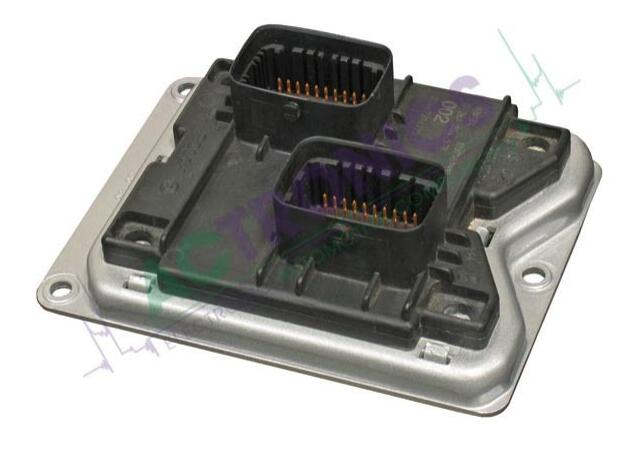Related Content:

In Motronic M1.5.5 there are 2 banks of repeated data.
First bank goes from 0000h to FFFFh.
Second bank goes from 10000h to 1FFFFh.
There are 2 checksums (one for each bank) located at FFF8h and 1FFF8h.
Both checksums are 16bit sums followed by their complement.
You can find this going to the end of each bank and finding the both 16bit numbers that  are complement of each other.
First one: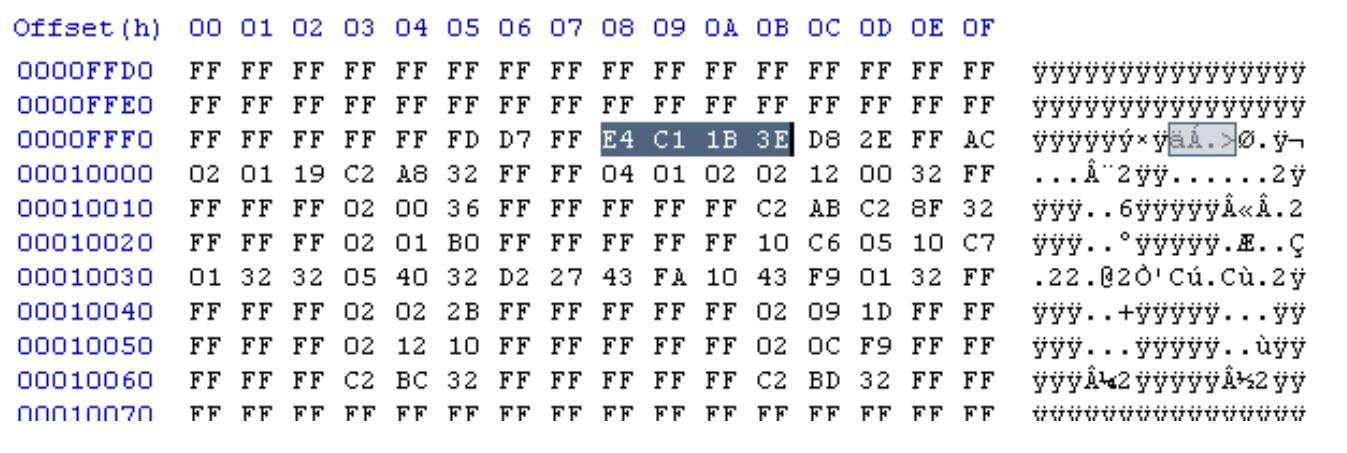Note that E4C1h + 1B3Eh = FFFFh
Second one: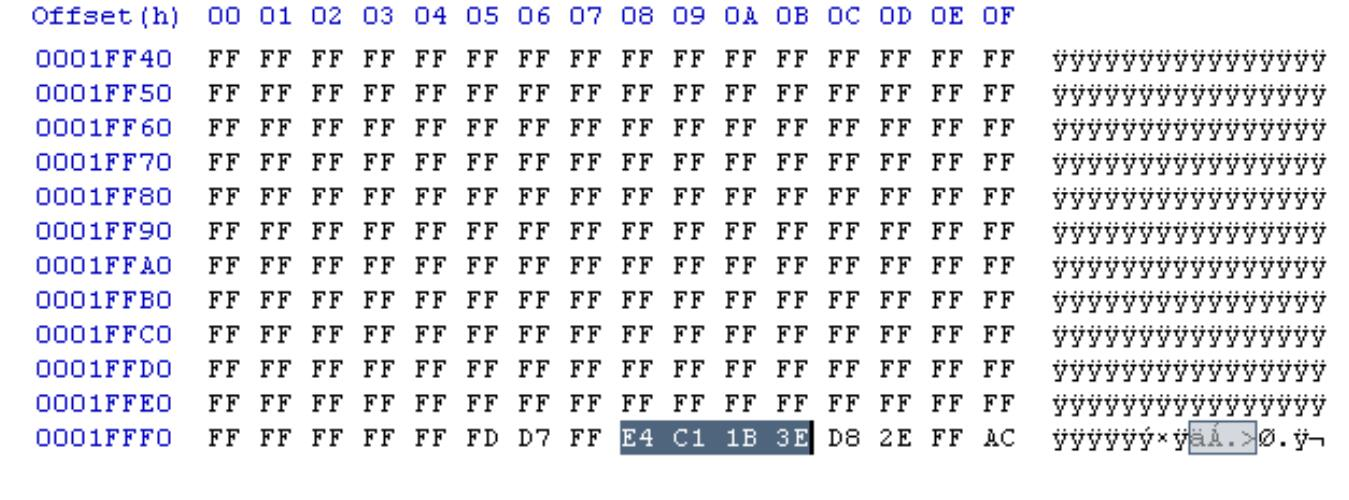Again, note that E4C1h + 1B3Eh = FFFFh (both banks are equal).

How to calculate the checksum for a modified file.
I’ll use HxD editor (http://mh-nexus.de/en/hxd/) but anything similar will do.
1.Set both checksums to 00 00 FF FF, that way the sum won’t change the data checksum.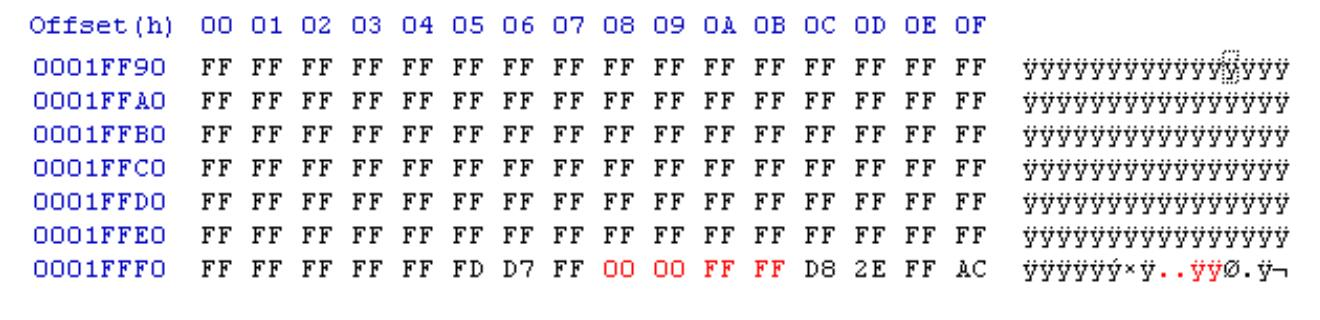2.Sum up all the 128kb using the checksum 16-bit function (under the Analysis menu).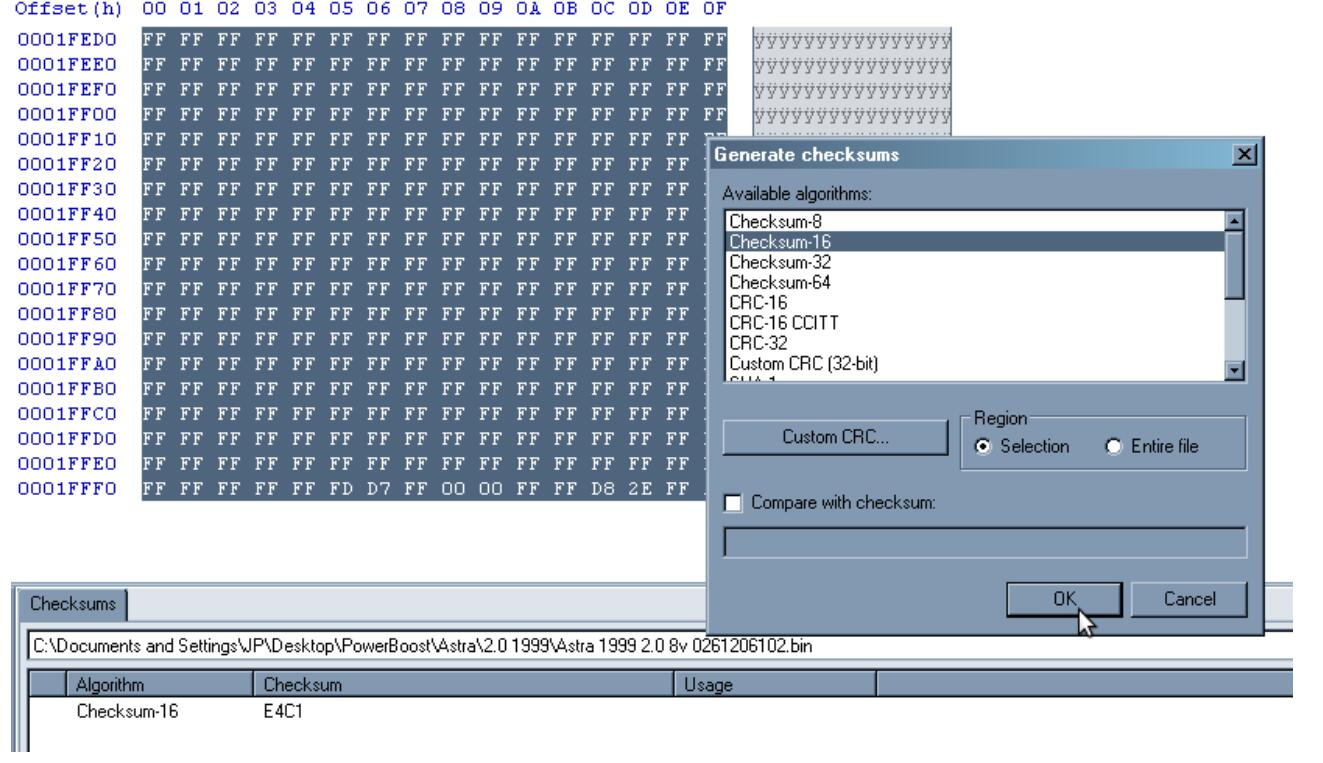3.Now, that value is the first part of both checksums. Using the calculator do FFFFh –Value to get the second checksum value.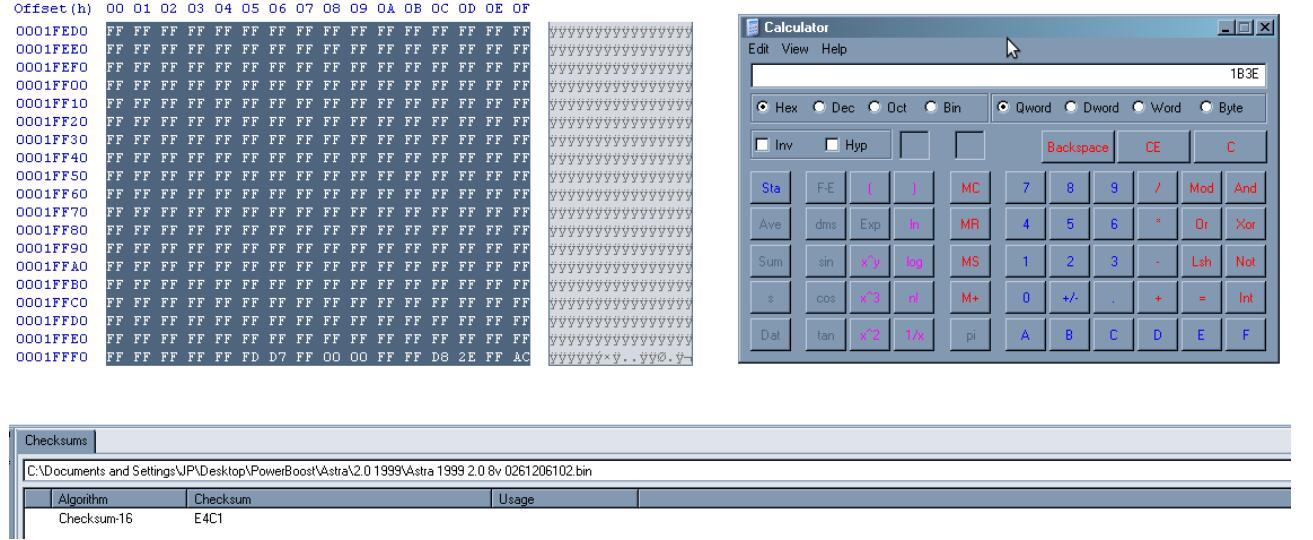4.Edit both checksums like (Value1,Value2).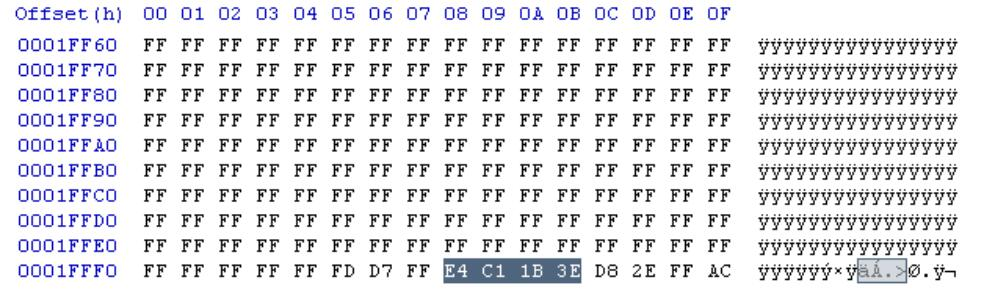5.Recheck everything by calculating again the 16bit sum of everything. That value should still be the same Value1.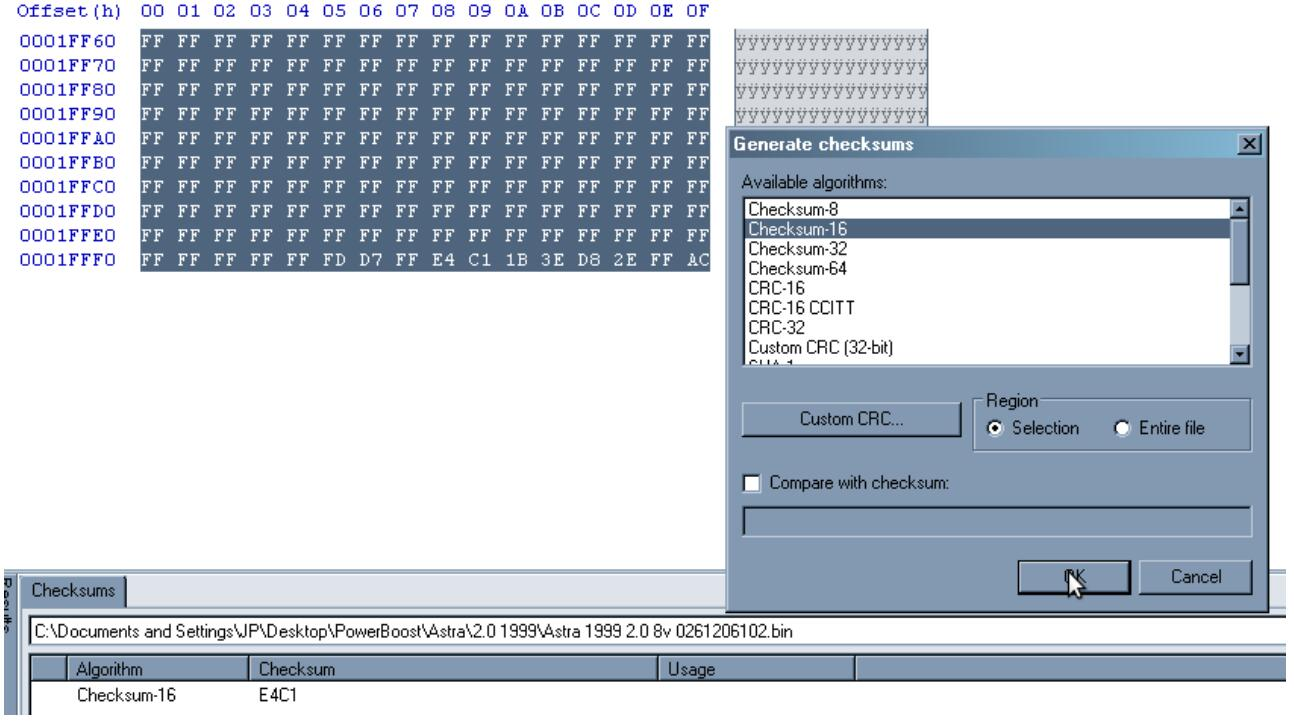(Visited 190 times, 1 visits today)